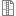# 17ABBRBL - Robotics in Medicine

Code Completion Credits Range Language
17ABBRBL KZ 2 1P+1L English
Garant předmětu:
Lecturer:
Tutor:
Supervisor:
Department of Biomedical Informatics
Synopsis:

Principles of robotics in medicine and laboratory technics - what kind of task is solving, synthesis of kinematics according to the task processed by robot - operational (surgical room), handling (laboratory), kinematics a dynamics of robot arm - computing methodology, verification of obtained models in Matlab environment, sensors and drives used by robots applicable in medicine, possible robot control paradigms - according human (operator) task.

Requirements:

pass test, solved task for control of kinematics chain and make program for it.

Syllabus of lectures:

1. Introduction to theory of robotics - kinematic joint, kinematic chain, degree of freedom, structural and kinematic schema. Kinematc of robot in homogenous coordinates system - homogenous transform, transform matrices, matrices of basic motions.

2. Position of point in different bodies system of kinematic chain, velocity matrix of body and velosity its point, acceleration matrix of body and

acceleration its point.

3. Kinematics of open chains - matrix of inverse motion, position, velosity and acceleration of end point to frame and others bodies.

4. Dynamics of open chains - approximation of mass distribution inside kinematics chain, potencial energy and kinetic energy of chain. Lagrangian equations of second type and their using for expression motion equations.

5. Computation of the Jacobians and their using for computation matrices C, D, and G. Equations of dynamics in matrix form.

6. Paradigm of kinematic control open chains.

7. Simulation of control robotic arm in Matlab.

Syllabus of tutorials:

1.Direct kinematics of the hand.

2.Dynamic model of hand.

3.Control of drives of 6-DOF arm to the desired location of the endpoint chain using a specialized software and hardware.

4.Controling of arm along the desired trajectory.

5.Transfer data (setpoint for the robotic system) with RFID Technology.

6.Realization of human-machine interface (with using biological signals).

Study Objective:

Principles of robotics in medicine and laboratory technics - what kind of task

is solving, synthesis of kinematics according to the task processed by robot -

operational (surgical room), handling (laboratory), kinematics a dynamics of

robot arm - computing methodology, verification of obtained models in Matlab

environment, sensors and drives used by robots applicable in medicine, possibleBook46.08 MBMatlab solution of arm dynamic 23.71 KB